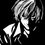# Another Interesting Series

Well, most of you would have definitely proved that the alternating sum of reciprocal of odd numbers equals $$\frac{\pi}{4}$$.

Here's a more generalized case of the series

$\sum_{k=0}^\infty (-1)^{k}\frac{2k+1}{(mk+n)(mk+m-n)} = \frac{\pi}{m^2}\csc\left(\frac{n\pi}{m}\right)$

Proof

It can be easily proved that

$\int_0^\infty \frac{x^{n-1}}{1+x^m} \mathrm{d}x = \frac{\pi}{m} \csc\left(\frac{n\pi}{m}\right)$

by making the substitution $y=\frac{1}{1+x^m}$ and then the integral converts to Beta Function and the final result follows by using Euler's Reflection Formula $\Gamma(z)\Gamma(1-z) = \frac{\pi}{\sin \pi z}$

Now, all that remains is to split the integral as

$\int_0^\infty \frac{x^{n-1}}{1+x^m} \mathrm{d}x = \int_0^1 \frac{x^{n-1}}{1+x^m} \mathrm{d}x + \int_1^\infty \frac{x^{n-1}}{1+x^m} \mathrm{d}x$

The first term evaluates to $\int_0^1 \frac{x^{n-1}}{1+x^m} \mathrm{d}x = \int_0^1 x^{n-1}\sum_{k=0}^\infty (-1)^k x^{mk}\ \mathrm{d}x = \sum_{k=0}^\infty \frac{(-1)^k}{mk+n}$

Similarly, the second term can be evaluated by making the following substitution : $y=\frac{1}{x}$ $\int_1^\infty \frac{x^{n-1}}{1+x^m} \mathrm{d}x = \int_0^1 \frac{y^{m-n-1}}{1+y^m} \mathrm{d}y = \sum_{k=0}^\infty \frac{(-1)^k}{mk+m-n}$

And thus the result follows. $\square$

Interesting part is that, the base case when $m=2,n=1$, you get the above mentioned well known series of alternating sum of reciprocal of odd numbers that is

$\sum_{k=0}^\infty \frac{(-1)^{k}}{2k+1} = \frac{\pi}{4}$

And I am sure, that you all must have got some other, perhaps more elegant, proof for the same. So, please do share it with all of us.Note by Kishlaya Jaiswal
5 years, 5 months ago

This discussion board is a place to discuss our Daily Challenges and the math and science related to those challenges. Explanations are more than just a solution — they should explain the steps and thinking strategies that you used to obtain the solution. Comments should further the discussion of math and science.

When posting on Brilliant:

• Use the emojis to react to an explanation, whether you're congratulating a job well done , or just really confused .
• Ask specific questions about the challenge or the steps in somebody's explanation. Well-posed questions can add a lot to the discussion, but posting "I don't understand!" doesn't help anyone.
• Try to contribute something new to the discussion, whether it is an extension, generalization or other idea related to the challenge.

MarkdownAppears as
*italics* or _italics_ italics
**bold** or __bold__ bold
- bulleted- list
• bulleted
• list
1. numbered2. list
1. numbered
2. list
Note: you must add a full line of space before and after lists for them to show up correctly
paragraph 1paragraph 2

paragraph 1

paragraph 2

[example link](https://brilliant.org)example link
> This is a quote
This is a quote
    # I indented these lines
# 4 spaces, and now they show
# up as a code block.

print "hello world"
# I indented these lines
# 4 spaces, and now they show
# up as a code block.

print "hello world"
MathAppears as
Remember to wrap math in $$ ... $$ or $ ... $ to ensure proper formatting.
2 \times 3 $2 \times 3$
2^{34} $2^{34}$
a_{i-1} $a_{i-1}$
\frac{2}{3} $\frac{2}{3}$
\sqrt{2} $\sqrt{2}$
\sum_{i=1}^3 $\sum_{i=1}^3$
\sin \theta $\sin \theta$
\boxed{123} $\boxed{123}$

Sort by:

what an amazing beautiful proof

- 5 years, 4 months ago

Thank You So Much.

- 5 years, 4 months ago